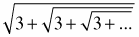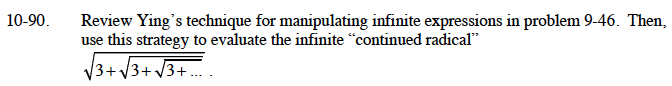### Home > CALC > Chapter 10 > Lesson 10.2.1 > Problem10-90

10-90.

Review Ying's technique for manipulating infinite expressions in problem 9-46. Then, use this strategy to evaluate the infinite "continued radical"Homework Help ✎$\text{Let }S=\sqrt{3+\sqrt{3+...}}$

Now solve for S using the Quadratic Formula.